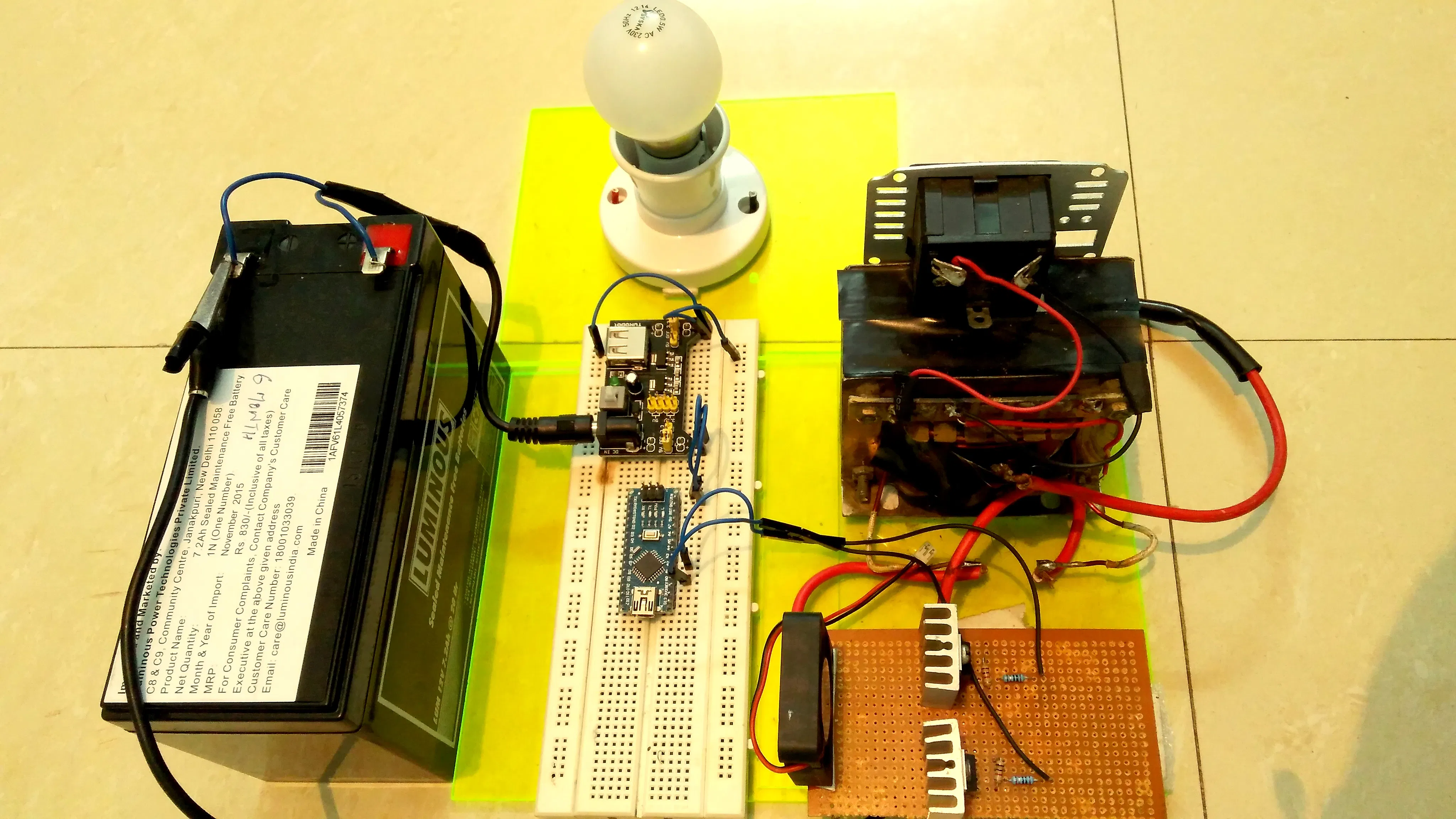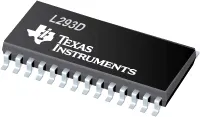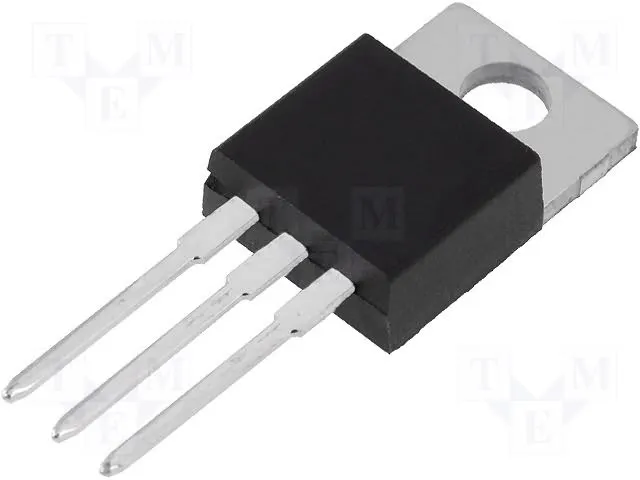# Make your own Power Inverter using Arduino

Step by step approach is followed so that any hobbyist or design engineer can have a better understanding of the basic concepts.

IntermediateShowcase (no instructions)41,362## Things used in this project

### Hardware componentsArduino Nano R3
×1
 IRF3205
×2Texas Instruments Dual H-Bridge motor drivers L293D
×1×1Linear Regulator (7805)
×1
 Transformer 12v to 220v / 600VA
×1

### Hand tools and fabrication machines

 MultimeterSoldering iron (generic)
 Wire
 Veroboard

## Schematics

### Inverter

It's complete schematic of the power inverter, drawn in EAGLE software

## Code

### Inverter arduino code

Arduino
Complete code of the driver stage.
```#include "TimerOne.h"               // include TimerOne.h
#define low_battery_voltage 10.2               // define high battery voltage limit as 14.2
#define high_battery_voltage 14.4               // define low battery voltage limit as 10.2
int dutycycle = 0;               // Initailize duty cylce variable as integer data type
int sense_value =0;               // Initialize sense_value variable to capture the adc reading of battery voltage (range from 0 to 1023)
float battery_voltage = 0.0;               // Initialize battery_voltage variable as a float data type to convert sense_value to actual battery voltage

void setup()               // Setup function
{
pinMode (9,OUTPUT);              // set pin 9 as an output pin for pwm
pinMode (10,OUTPUT);             // set pin 10 as an output pin for pwm
Timer1.initialize(20000);             // Initailize timer1 time period as 20 milli second (50 Hz frequency)
Timer1.attachInterrupt(battery_voltage_measurement);      // battery_voltage_measurement function will be executed every 20 milli second using timer 1 overflow interrupt
TCCR1A = (TCCR1A & 0x0F) | 0xB0 ;             // set pin 10 inverted of pin 9
}

void battery_voltage_measurement()               // battery_voltage_measurement function starts
{ sense_value = (analogRead(A0));               // read battery voltage on pin A0 & capture that value in sense_value variable
// {warning - arduino pin accept only upto 5v so don't forget to map upper
//  battery volatge i.e 14.2v to 5v using voltage divider resistor network}

battery_voltage = sense_value * (14.4/1023.0);               // convert sense_value (0 to 1023) to range (0 to 14.2)
if(battery_voltage < 14.4 && battery_voltage > 10.2) // if battery voltage is under limit i.e between 10.2 and 14.2 then dutycycle will be 150
{
dutycycle = 300;
}

else if(battery_voltage < 10.2 || battery_voltage > 14.4)              // if battery voltage is below 10.2v or above 14.2v ,
{
dutycycle = 0;         //set the duty cycle to 0 and inverter will go in cutoff mode
}

}              // battery_voltage_measurement function ends

void loop()              // loop function starts
{
Timer1.pwm(9,dutycycle,20000);              // Timer1.pwm function takes argument as (pin no. , dutycycle , time period)
Timer1.pwm(10,1023-dutycycle,20000);

}               // loop function ends

```

## Credits

### user534361260

1 project • 8 followers